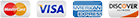# Convert XLSX to PDF Command Line

Spreadsheet programs are perfect for manipulating tabular data but there is often a need to convert their contents into other formats such as PDF for easy sharing, presentation or archiving of data. Converting those files by hand, particularly if there are a huge amount of them or if the conversion has to take place on a regular schedule, is an error-prone waste of valuable time and a task that cries out for automation.

Our Total Excel Converter X software is the logical tool to use when you are looking to convert XLSX to PDF via command line at scale. The program takes all the functionality users are used to from Total Excel Converter and adds an extra dimension of power by including support for server based conversion and integration into web applications via ActiveX.

Whether you are looking for a command line XLSX converter or a server XLSX converter that can be called directly from your own application, Total Excel Converter X has you covered. Here are just some of the advanced features available:

• Take advantage of a huge range of input and export formats. All current versions of Excel are supported in addition to OpenOffice, XML and SQL.
• Take control of renaming output files with the powerful built-in renamer.
• Combine several spreadsheets into one file with ease.
• Define your own header and footer settings by adding elements such as dates, file names or page counters to your output files.
• Convert common tasks to executable BAT files if necessary via a GUI.
• Leverage the power of security options when choosing PDF as your output format.

When you are looking to take advantage of the full power of a programmatic conversion solution, the last thing you want is to be interrupted by a stream of system messages.

Total Excel Converter X runs seamlessly via the command line or when integrated with your applications so you do not have to sit there monitoring it as it runs. Any error messages encountered will be logged silently to an easily readable log file where you can inspect them later at your leisure in order to troubleshoot.

We are confident that Total Excel Converter X will introduce a brand new level of programmatic power to your document conversion workflow and encourage you to take it for a test run prior to purchasing. Download your free trial copy today and discover its full range of features for yourself!## Examples of Total Excel ConverterX

### Convert excel files With TotalExcelConverterX and .NET

```string src="C:\\test\\Source.xlsx";
string dest="C:\\test\\Dest.PDF";

ExcelConverterX Cnv = new ExcelConverterX();
Cnv.Convert(src, dest, "-c PDF -log c:\\test\\Excel.log");

MessageBox.Show("Convert complete!");
```

### Convert Excel Files On Web Servers With Total Excel ConverterX

```dim C
Set C=CreateObject("ExcelConverter.ExcelConverterX")
C.Convert "c:\test\source.xlsx", "c:\test\dest.pdf", "-cPDF -log c:\test\Excel.log"
Response.Write C.ErrorMessage
set C = nothing
```
Example2 ASP: directly stream the resulting PDF
```dim C
Set C=CreateObject("ExcelConverter.ExcelConverterX")
Response.Clear
Response.BinaryWrite c.ConvertToStream("C:\www\ASP\Source.xlsx", "C:\www\ASP", "-cpdf  -log c:\html.log")
set C = nothing
```

### Convert Excel Spreadsheets by PHP With Total Excel ConverterX

```\$src="C:\\test\\test.XLS";
\$dest="C:\\test\\test.CSV";
\$c= new COM("ExcelConverter.ExcelConverterX");
\$c->convert(\$src,\$dest, "-c csv  -log c:\\test\\xls.log");
if (file_exists(\$dest)) echo "OK"; else echo "fail:".\$c->ErrorMessage;
```

### Convert Excel Spreadsheets By Ruby With Total Excel ConverterX

```require 'win32ole'
c = WIN32OLE.new('ExcelConverter.ExcelConverterX')

src="C:\\test\\test.xlsx";
dest="C:\\test\\test.pdf";

c.convert(src,dest, "-c PDF -log c:\\test\\Excel.log");

if not File.exist?(dest)
puts c.ErrorMessage
end

```

### Convert Excel Spreadsheets by Python With Total Excel ConverterX

```import win32com.client
import os.path

c = win32com.client.Dispatch("ExcelConverter.ExcelConverterX")

src="C:\\test\\test.xlsx";
dest="C:\\test\\test.pdf";

c.convert(src, dest, "-c PDF -log c:\\test\\Excel.log");

if not os.path.exists(file_path):
print(c.ErrorMessage)
```

### Convert Excel files With Pascal and Total Excel ConverterX

```uses Dialogs, Vcl.OleAuto;

var
c: OleVariant;
begin
c:=CreateOleObject('ExcelConverter.ExcelConverterX');
C.Convert('c:\test\source.xlsx', 'c:\test\dest.pdf', '-cPDF -log c:\test\Excel.log');
IF c.ErrorMessage<> Then
ShowMessage(c.ErrorMessage);
end;
```

### Convert Excel and ODS Files On Web Servers With Total Excel ConverterX

```var c = new ActiveXObject("ExcelConverter.ExcelConverterX");
c.Convert("C:\\test\\source.xlsx", "C:\\test\\dest.pdf", "-c PDF");
if (c.ErrorMessage!="")
```

### Convert Excel Spreadsheets by Perl With Total Excel ConverterX

```use Win32::OLE;

my \$src="C:\\test\\test.XLS";
my \$dest="C:\\test\\test.CSV";

my \$c = CreateObject Win32::OLE 'ExcelConverter.ExcelConverterX';
\$c->convert(\$src,\$dest, "-c csv  -log c:\\test\\xls.log");
print \$c->ErrorMessage if -e \$dest;
```

### Latest News

• New Total PDF Printer has been released. Now you can print a folder of PDF files and then move the files to a different folder (-msuccess).
• We have fixed an issue in Coolutils Converter where some messages in HTML were not showing correctly.
• New Total HTML Converter has got an update with a few important bug fixes (large radius, paged layout).
• New Total Mail Converter Pro offers enhanced option to fit extra wide tables from emails to PDF files.
• New PDF Combine 7.0 is live. New options for creating combined PDF scans, e-books and songbooks.
• New Total Excel Converter 6.0 is live. Convert XLS with with charts.